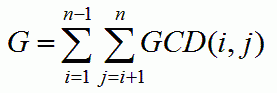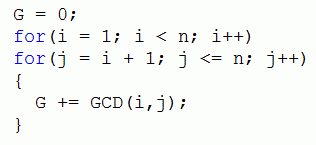favorite We need a little bit of your help to keep things running, click on this banner to learn more
Competitions

# GCD Extreme II

For a given n calculate the value of G, whereHere GCD(i, j) means the greatest common divisor of integers i and j.

For those who have trouble understanding summation notation, the meaning of G is given in the following code:Here GCD() is a function that finds the greatest common divisor of the two input numbers.

#### Input

Consists of no more than 20000 lines. Each line contains an integer n (1 < n < 4000001). The meaning of n is given in the problem statement. Input is terminated by a line containing a single zero and should not be processed.

#### Output

For each input number n print in a separate line the corresponding value of G. The value of G fits in a 64-bit signed integer.

Time limit 2 seconds
Memory limit 128 MiB
Input example #1
10
100
200000
0

Output example #1
67
13015
143295493160